## Hysteresis Mitigation in Current Sensor ICs Using Ferromagnetic Cores

By Georges El Bacha, Shaun Milano, and Jeff Viola
Allegro MicroSystems, LLC

Introduction

Traditional open loop current sensor ICs, like the Allegro ACS758CB and ACS770CB families, have ferromagnetic cores that act as magnetic concentrators. They concentrate the magnetic flux density, the B field, generated by current flowing through a conductor onto the Hall-effect sensor IC as illustrated in Figure 1.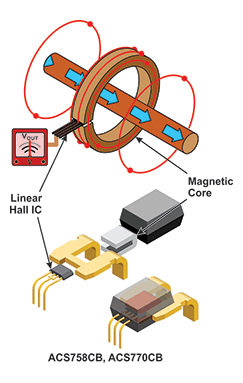Figure 1: Sensing Current Using a Hall Sensor IC and Magnetic Core

The Hall-effect sensor IC has a Hall element, a transducer, that converts the B field perpendicular to the Hall element into a voltage. This Hall sensor voltage is directly proportional to the B field. The B field is also proportional to the magnitude of the current in the conductor, and the Hall sensor output voltage is therefore directly proportional to the magnitude of the current flowing in the conductor. In this way, very accurate current sensors can be made with a Hall-effect sensor and concentrating core.

Without the core, the B field around the conductor would be small and difficult to measure accurately. Cores can amplify the field 20x or more and are therefore extremely valuable for improving sensor accuracy and resolution. There are several other advantages to measuring current in this fashion, such as Galvanic Isolation, very low power losses and low heat generation. The one disadvantage with using a ferromagnetic material as a concentrating core is magnetic hysteresis.

What is Magnetic Hysteresis?

Magnetic hysteresis is measured by taking a piece of the core material and generating a B-H curve. An external magnetic field (H) is applied to the material and then the magnetic flux density (B) ‘inside’ the material is measured. A family of curves for a permanent magnet or “hard” material is shown below in Figure 2. Permanent magnets are not used as magnetic cores but help to illustrate how magnetic hysteresis works. When a large field is applied, the magnetic material is magnetized; when the magnetizing field (H) is removed, a permanent magnetic field exists around the material with flux density (B) shown in Figure 2.

The field generated by the permanent magnet depends not only on the material but also on how hard it was magnetized. In other words, it depends on how much H field was applied during magnetization. By applying different magnetization fields (H), a family of curves can be generated as shown in Figure 2.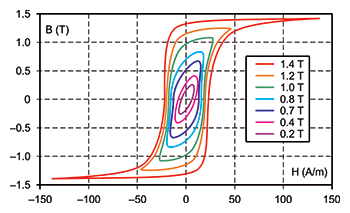Figure 2: B versus H Family of Curves

Ferromagnetic materials are materials that magnetize or are attracted to permanent magnets. They have high magnetic permeability and all of them have domains that line up in the presence of a magnetic field (refer to Figure 3). Domains that are loosely held revert back to a random orientation after the applied magnetic field is removed. These are called “soft” materials and are desirable for use as cores. Not all of the domains revert back to random orientation, and that is how the material becomes slightly magnetized. This is the ‘magnetic remanence’ and is the hysteresis of the material. Permanent magnet domains remain locked in the same orientation as the magnetizing field and are therefore “hard” materials.

A soft ferromagnetic material with low hysteresis is desired when choosing a core material for current sensing applications, as illustrated in Figures 3 and 4.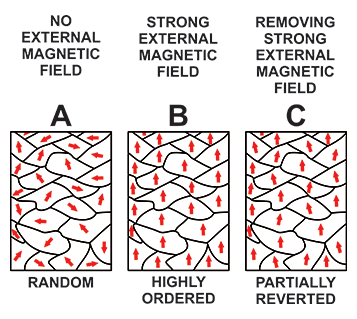Figure 3: Magnetic Domains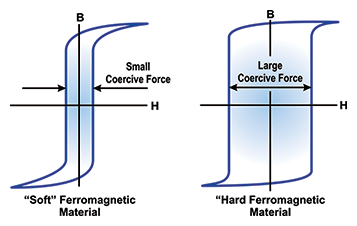Figure 4: Soft and Hard B versus H Loops

When a Hall current sensor IC is placed in the gap of the core and no current is flowing, the device output voltage should indicate zero amps. Magnetic hysteresis in the core will retain a magnetic field after current flows in the conductor, because the current flow generates an applied field and will magnetize the core material. When the current is no longer flowing, the Hall sensor will measure a non-zero current, depending on the level of magnetization of the core material. This results in some error in the zero amp reading and is, therefore, undesirable.

Soft versus Hard Material

Allegro CA/CB packaged current sensor ICs employ a soft ferromagnetic core material. These soft magnetic materials have much less remanence or hysteresis. To explain by way of example, the most common plain steel for general use is 1020 steel. 1020 can retain 30 Gauss (G) in its hot rolled state and considerably more in its cold-rolled state. The SiFe material used in the Allegro CB package retains on the order of 2 G. So the material is optimized for use as a core for current sensing, as it will minimize the zero current output error of the Hall sensor.

Magnetic Hysteresis Effect on the ACS758CB Current Sensor

The ACS758CB Quiescent Output Voltage (VOUTQ ) is the output of the current sensor IC when the primary current is zero. For bidirectional devices, it nominally remains at VCC ⁄ 2. VCC = 5 V translates into an ideal VOUTQ = 2.5 V.

As described earlier, the core used inside the current sensor IC has a remanence that impacts VOUTQ level after a current has been applied to the sensor. The following convention will be used for the remaining of the article.

• Positive Quiescent Output Voltage (VOUTQP): Measured output voltage after the ‘Maximum Positive’ applied current has been injected in the current sensor IC then reduced to 0 A.
• Negative Quiescent Output Voltage (VOUTQN): Measured output voltage after the ‘Maximum Negative’ applied current has been injected in the current sensor IC then reduced to 0 A.
• Ideal Quiescent Output Voltage (VOUTQI): Average of VOUTQP and VOUTQN where Maximum Positive and Negative current have the same magnitude.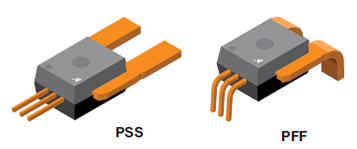Figure 5: Allegro CA/CB Packaged Current Sensor ICs

Figure 6 below, illustrates Quiescent Output Voltage after different current pulses were applied to a ACS758LKCB-150B (150 A bidirectional version of the sensor). Current sensor IC output was recorded after each current pulse was reduced to 0 A. Maximum application current was set to ±130 A during measurements. To generate these plots a 130 A pulse was applied to the sensor  followed by a series of negative current pulses ranging from -3 A  to -130 A . This was followed by a series of positive current pulses ranging from 3 A  to 130 A . Similar measurements were repeated with maximum current amplitudes of 90 A  and 50 A .

Remanence of the ACS758 core causes the VOUTQ to vary depending on the magnitude and polarity of the injected current. The 130 A hysteresis loop (outer most curve in green) has a maximum VOUTQP of 2.5032 V (after 130 A pulse) and a minimum VOUTQN of 2.4932 V (after -130 A pulse) with a middle point VOUTQI of 2.4982 V . This a difference of 10 mV or ±5 mV variation from VOUTQI.

With a sensitivity of 13.3 mV/A for a 150 A bidirectional sensor, this gives us a Magnetic Offset or Hysteresis of 5 mV/13.3 mV/A = ±375.9 mA. This is only 0.289% of the 130 A maximum application current used during our measurement. Typically the ACS758CB has a magnetic offset of ±250 mA. A device with a larger magnetic offset was used in this example to illustrate a near worst case scenario.

ACS758 150 A Bidirectional Hysteresis Loops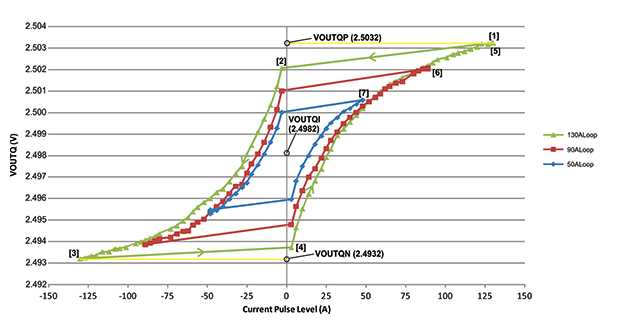Figure 6: ACS758 Family Hysteresis Plot

How do we Mitigate Magnetic Hysteresis?

Method One

The easiest thing we can do is simply cut the full Peak to Peak value of hysteresis in half. This can be done by applying maximum positive and negative application current, recording VOUTQP, VOUTQN and calculating VOUTQI. VOUTQI should be stored in system memory and used as the expected zero current output voltage (refer to Figure 7).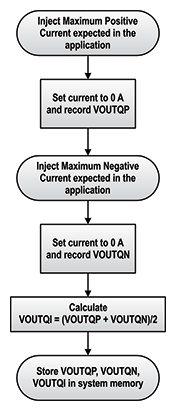Figure 7: How to Measure VOUTQP, VOUTQN, and VOUTQI

To use the data measured in Figure 6 as our example, for a ±130 A maximum application current, VOUTQP = 2.5032 V, VOUTQN = 2.4932 V, VOUTQI = (2.5032+2.4932)/2 = 2.4982 V. By simply using this VOUTQI as the expected zero current output voltage we can never be off by more than ±5 mV or ±375.9 mA.

Before introducing the next compensation method, the Coercive Current needs to be defined. Coercive Current is the current level required to reduce the magnetization of that material to near zero, after the sensor has been exposed to the maximum application current. For example in Figure 8, after a 130 A pulse, VOUTQP = 2.5032 V. A -25 A current pulse is required to reduce the ACS758 magnetization to near zero. This will cause VOUTQ to be near VOUTQI = 2.4982 V, the ideal VOUTQ. The Coercive Current in a system where the maximum application current is ±130 A is ±25 A.

ACS758 150 A Bidirectional Hysteresis Loops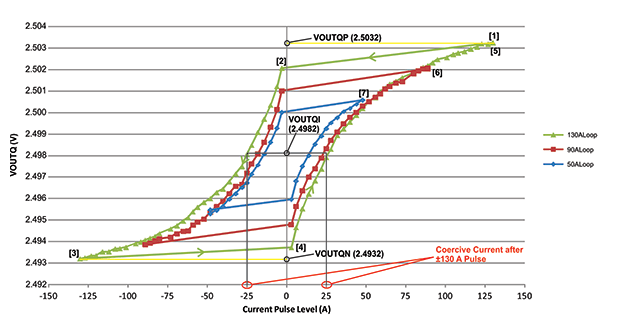Figure 8: Coercive Current Value for a ±130 A Maximum Application Current System

Method Two

As in method one, apply both positive and negative maximum currents and record VOUTQP and VOUTQN then calculate VOUTQI during system calibration. During operation, Current Polarity and Magnitude should be tracked.

If the current polarity did not change and the current magnitude was less than or equal to the last largest measured current then VOUTQ does not need to be updated.

If the current polarity changed and the magnitude is near the coercive value, then VOUTQI should be used.

If the current polarity changed and the magnitude is significantly larger than the coercive value, then use VOUTQP (for positive current) or VOUTQN (for negative current).

If the current polarity changed and the magnitude is significantly below the coercive value then, VOUTQ should remain at its present value.

Depending on the application, the user can select limits considered ‘significantly’ bigger and smaller than the Coercive value. These limits form the Coercive Window, Figure 9.

A detailed block diagram of the Method Two algorithm can be found on Figure 10.

In our example where the maximum application current is ±130 A, while Method One yielded a maximum error of ±5 mV Method Two will yield a maximum error of ±2.5 mV.

ACS758 150 A Bidirectional Hysteresis Loops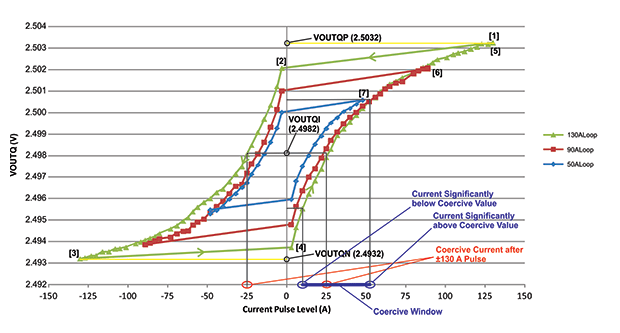Figure 9: Example of Current Values Selected to Form a Coercive Window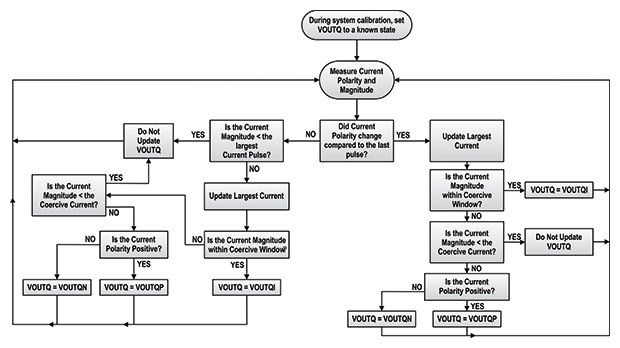Figure 10: Method Two Algorithm

Conclusion

Current sensor ICs using ferromagnetic concentrators have magnetic hysteresis. In the case of the ACS758CB and ACS770CB the magnetic hysteresis is generally small and with proper system and software development it can be significantly reduced.